# Specify Custom Signal Objective with Uncertain Variable (GUI)

This example shows how to specify a custom objective function for a model signal. You calculate the objective function value using a variable that models parameter uncertainty.

### Competitive Population Dynamics Model

The Simulink® model sdoPopulation models a simple two-organism ecology using the competitive Lotka-Volterra equations:•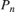is the population size of the n-th organism.

•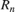is the inherent per capita growth rate of each organism.

•is the competitive delay for each organism.

•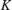is the carrying capacity of the organism environment.

•is the proximity of the two populations and how strongly they affect each other.

The model uses normalized units.

Open the model.

open_system('sdoPopulation')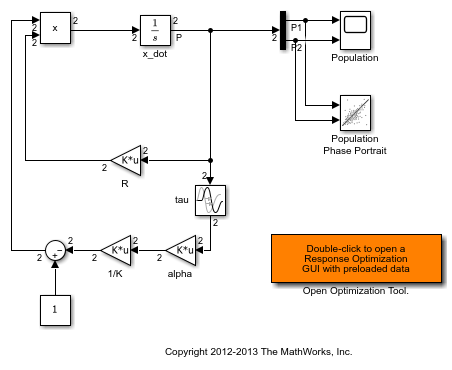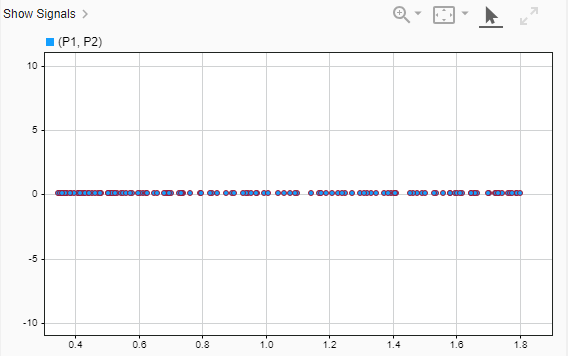The two-dimensional signal, P, models the population sizes for P1 (first element) and P2 (second element). The model is initially configured with one organism, P1, dominating the ecology. The Population scope shows the P1 population oscillating between high and low values, while P2 is constant at 0.1. The Population Phase Portrait block shows the population sizes of the two organisms in relation to each other.

### Population Stabilization Design Problem

Tune the,, andvalues to meet the following design requirements.

• Minimize the population range, that is, the maximum difference between P1 and P2.

• Stabilize P1 and P2, that is, ensure that neither organism population dies off or grows extremely large.

You must tune the parameters for different values of the carrying capacity,. This ensures robustness to environment carrying-capacity uncertainty.

### Open Response Optimizer

Double-click the Open Optimization Tool block in the model to open a pre-configured Response Optimizer session. The session specifies the following variables:

• DesignVars - Design variables set for the,, andmodel parameters.

• K_unc - Uncertain parameter modeling the carrying capacity of the organism environment (). K_unc specifies the nominal value and two sample values.

• P1 and P2 - Logged signals representing the populations of the two organisms.

### Specify Custom Signal Objective Function

Specify a custom requirement to minimize the maximum difference between the two population sizes. Apply this requirement to the P1 and P2 model signals.

1. Open the Create Requirement dialog box. In the New list, select Custom Requirement.

2. Specify the following in the Create Requirement dialog box:

• Name - Enter PopulationRange.

• Type - Select Minimize the function output from the list.

• Function - Enter @sdoPopulation_PopRange. For more information about this function, see Custom Signal Objective Function Details.

• Select Signals and Systems to Bound (Optional) - Select the P1 and P2 check boxes.3. Click OK.

A new variable, PopulationRange, appears in the Response Optimizer browser.

### Custom Signal Objective Function Details

PopulationRange uses the sdoPopulation_PopRange function. This function computes the maximum difference between the populations, across different environment carrying capacity values. By minimizing this value, you can achieve both design goals. The function is called by the optimizer at each iteration step.

To view the function, type edit sdoPopulation_PopRange. The following discusses details of this function.

Input/Output

The function accepts data, a structure with the following fields:

• DesignVars - Current iteration values of,, and.

• Nominal - Logged signal data, obtained by simulating the model using parameter values specified by data.DesignVars and nominal values for all other parameters. The Nominal field is itself a structure with fields for each logged signal. The field names are the logged signal names. The custom requirement uses the logged signals, P1 and P2. Therefore, data.Nominal.P1 and data.Nominal.P2 are timeseries objects corresponding to P1 and P2.

• Uncertain - Logged signal data, obtained by simulating the model using the sample values of the uncertain variable K_unc. The Uncertain field is a vector of N structures, where N is the number of sample values specified for K_unc. Each element of this vector is similar to data.Nominal and contains simulation results obtained from a corresponding sample value specified for K_unc.

The function returns the maximum difference between the population sizes across different carrying capacities. The following code snippet in the function performs this action:

val = max(maxP(1)-minP(2),maxP(2)-minP(1));

Data Time Range

When computing the design goals, discard the initial population growth data to eliminate biases from the initial-condition. The following code snippet in the function performs this action:

%Get the population data tMin = 5; %Ignore signal values prior to this time iTime = data.Nominal.P1.Time > tMin; sigData = [data.Nominal.P1.Data(iTime), data.Nominal.P2.Data(iTime)];

iTime represents the time interval of interest, and the columns of sigData contain P1 and P2 data for this interval.

Optimization for Different Values of Carrying Capacity

The function includes the effects of varying the carrying capacity by iterating through the elements of data.Uncertain. The following code snippet in the function performs this action:

... for ct=1:numel(data.Uncertain) iTime = data.Uncertain(ct).P1.Time > tMin; sigData = [data.Uncertain(ct).P1.Data(iTime), data.Uncertain(ct).P2.Data(iTime)];
 maxP = max([maxP; max(sigData)]); %Update maximum if new signals are bigger minP = min([minP; min(sigData)]); %Update minimum if new signals are smaller end ...

The maximum and minimal populations are obtained across all the simulations contained in data.Uncertain.

### Optimize Design

Click Optimize.

The optimization converges after a number of iterations.The P1,P2 plot shows the population dynamics, with the first organism population in blue and the second organism population in red. The dotted lines indicate the population dynamics for different environment capacity values. The PopulationRange plot shows that the maximum difference between the two organism populations reduces over time.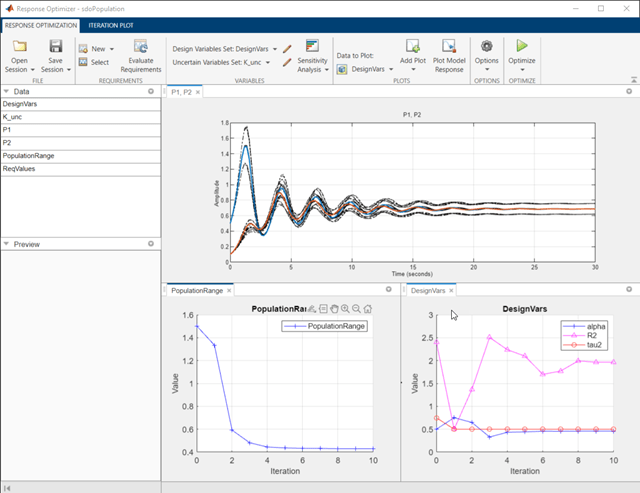The Population Phase Portrait block shows the populations initially varying, but they eventually converge to stable population sizes.% Close the model. bdclose('sdoPopulation')